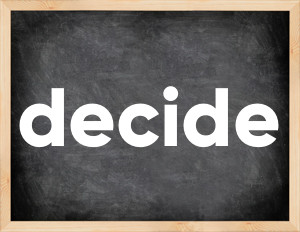# Decide past tenseThe English verb 'decide' is pronounced as [dɪˈsaɪd].
Related to: regular verbs.
3 forms of verb decide: Infinitive (decide), Past Simple - (decided), Past Participle - (decided).

## Here are the past tense forms of the verb decide

👉 Forms of verb decide in future and past simple and past participle.
❓ What is the past tense of decide.

## Decide: Past, Present, and Participle Forms

Base Form Past Simple Past Participle
decide [dɪˈsaɪd]

decided [dɪˈsaɪ.dɪd]

decided [dɪˈsaɪ.dɪd]

## What are the 2nd and 3rd forms of the verb decide?

🎓 What are the past simple, future simple, present perfect, past perfect, and future perfect forms of the base form (infinitive) 'decide'?

### Learn the three forms of the English verb 'decide'

• the first form (V1) is 'decide' used in present simple and future simple tenses.
• the second form (V2) is 'decided' used in past simple tense.
• the third form (V3) is 'decided' used in present perfect and past perfect tenses.

## What are the past tense and past participle of decide?

The past tense and past participle of decide are: decide in past simple is decided, and past participle is decided.

### What is the past tense of decide?

The past tense of the verb "decide" is "decided", and the past participle is "decided".

### Verb Tenses

Past simple — decide in past simple decided (V2).
Future simple — decide in future simple is decide (will + V1).
Present Perfect — decide in present perfect tense is decided (have/has + V3).
Past Perfect — decide in past perfect tense is decided (had + V3).

### decide regular or irregular verb?

👉 Is 'decide' a regular or irregular verb? The verb 'decide' is regular verb.

## Examples of Verb decide in Sentences

•   Let them decide what they can handle (Present Simple)
•   Perhaps he decided he needed a significant raise (Past Simple)
•   The King has decided on a bringing in a new Queen (Present Perfect)
•   You can't just decide for me (Present Simple)
•   They've already decided, so we can move further (Present Perfect)
•   I don't know what's the best answer, he is deciding on that right now (Present Continuous)
•   I was deciding which of these girls I would marry (Past Continuous)
•   They have decided to put aside that issues (Present Perfect)
•   We have decided to keep silence (Present Perfect)
•   Think they will decide later, let's have some rest (Future Simple)

Along with decide, words are popular spring and prefer.

Verbs by letter: , , , , , , , , , , , , , , , , , , , , , , , , .